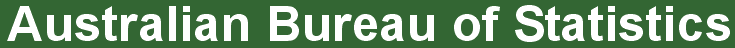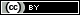ABS Home
 Statistical Language - Measures of ErrorMeasures of Error

Recommended: First read Types of Error

Why do we measure error?

Error is expected in a data collection process, particularly if the data is obtained from a sample survey. Although non-sampling error is difficult to measure, sampling error can be measured to give an indication of the accuracy of any estimate value for the population. This assists users to make informed decisions about whether the statistics are suited to their needs.

How do we measure error?

Two common measures of error include the standard error and the relative standard error.

Standard Error (SE) is a measure of the variation between any estimated population value that is based on a sample rather than true value for the population. As the standard error of an estimated value generally increases with the size of the estimate, a large standard error may not necessarily result in an unreliable estimate. Therefore it is often better to compare the error in relation to the size of the estimate.

Relative Standard Error (RSE) is the standard error expressed as a proportion of an estimated value. It is usually displayed as a percentage. RSEs are a useful measure as they provide an indication of the relative size of the error likely to have occurred due to sampling. A high RSE indicates less confidence that an estimated value is close to the true population value.

Where published statistics contain an indication of the RSEs they can be used to compare statistics from different studies of the same population.

What can measures of error tell us?

The standard error can be used to construct a confidence interval.

A confidence interval is a range in which it is estimated the true population value lies. Confidence intervals of different sizes can be created to represent different levels of confidence that the true population value will lie within a particular range. A common confidence interval used in statistics is the 95% confidence interval. In a 'normal distribution', the 95% confidence interval is measured by two standard errors either side of the estimate.

Further information:

ABS:
What is a Standard Error and Relative Standard Error?

 Privacy | Disclaimer | Feedback || © Copyright| Sitemap| Online Security